# Intel MKL-DNN: Part 2 – Code Build and Walkthrough

By | Updated 10 May 2017Introduction

In Part 1 we introduced Intel® Math Kernel Library for Deep Neural Networks (Intel® MKL-DNN), an open source performance library for deep learning applications. Detailed steps were provided on how to install the library components on a computer with an Intel processor supporting Intel® Advanced Vector Extensions 2 (Intel® AVX2) and running the Ubuntu* operating system. Details on how to build the C and C++ code examples from the command line were also covered in Part 1.

advertisements

In Part 2 we will explore how to configure an integrated development environment (IDE) to build the C++ code example, and provide a code walkthrough based on the AlexNet* deep learning topology. In this tutorial we’ll be working with the Eclipse Neon* IDE with the C/C++ Development Tools (CDT). (If your system does not already have Eclipse* installed you can follow the directions on the Ubuntu Handbook site, specifying the Oracle Java* 8 and Eclipse IDE for C/C++ Developers options.)

Building the C++ Example in Eclipse IDE

This section describes how to create a new project in Eclipse and import the Intel MKL-DNN C++ example code.

advertisements

Create a new project in Eclipse:

• Start Eclipse.
• Click New in the upper left-hand corner of screen.
• In the Select a wizard screen, select C++ Project and then click Next (Figure 1).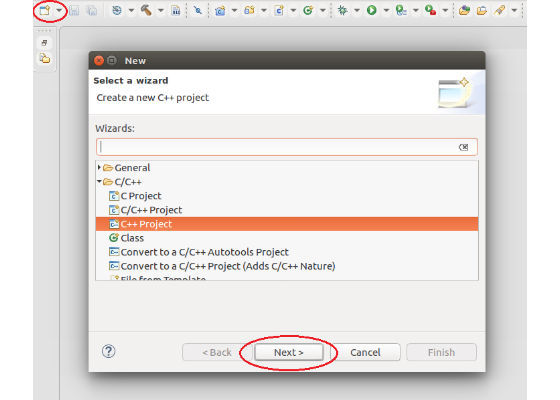Figure 1.Create a new C++ project in Eclipse.

• Enter simple_net for the project name. For the project type select Executable, Empty Project. For toolchain select Linux GCC. Click Next.
• In the Select Configurations screen, click Advanced Settings.

Enable C++11 for the project:

advertisements
• In the Properties screen, expand the C/C++ Build option in the menu tree and then select Settings.
• In the Tool Settings tab, select GCC C++ Compiler, and then Miscellaneous.
• In the Other flags box add -std=c++11 to the end of existing string separated by a space (Figure 2).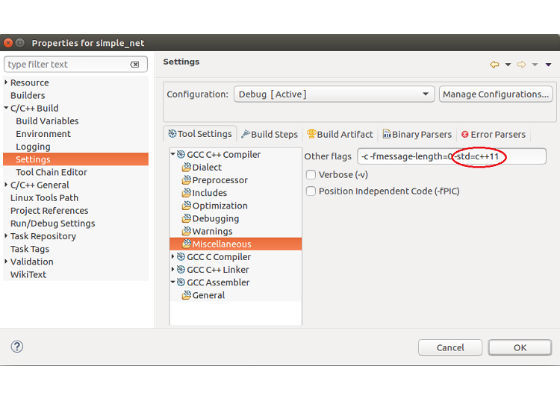Figure 2. Enable C++11 for the project (1 of 2).

• In the Properties screen, expand the C/C++ General and then select Preprocessor Include Paths, Macros etc.
• Select the Providers tab and then select the compiler you are using (for example, CDT GCC Built-in Compiler Settings).
• Locate the field named Command to get compiler specs: and add -std=c++11. The command should look similar to this when finished:

“\${COMMAND} \${FLAGS} -E -P -v -dD “\${INPUTS}” -std=c++11”.

advertisements
• Click Apply and then OK (Figure 3).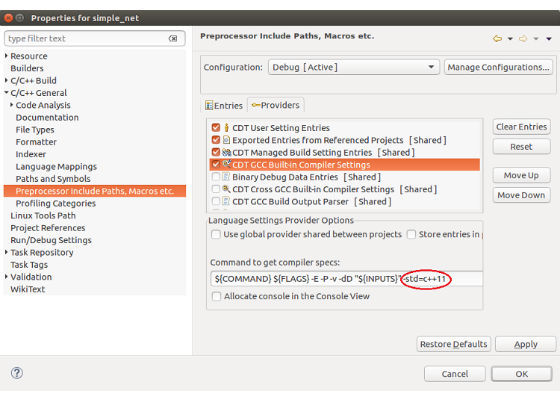Figure 3. Enable C++11 for the project (2 of 2).

Add library to linker settings:

• In the Properties screen, expand the C/C++ Build option in the menu tree and then select Settings.
• In the Tool Settings tab, select GCC C++ Linker, and then Libraries.
• Under the Libraries (l) section click Add.
• Enter mkldnn and then click OK (Figure 4).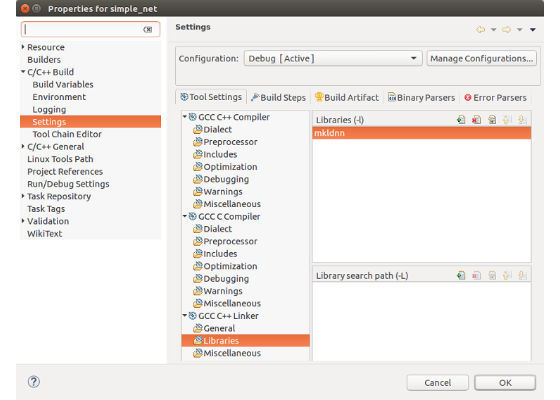advertisements

Figure 4. Add library to linker settings.

Finish creating the project:

• Click OK at the bottom of the Properties screen.
• Click Finish at the bottom of the C++ Project screen.

Add the C++ source file (note: at this point the simple_net project should appear in Project Explorer):

• Right-click the project name in Project Explorer and select New, Source Folder. Enter src for the folder name and then click Finish.
• Right-click the src folder in Project Explorer and select Import…
• In the Import screen, expand the General folder and then highlight File System. Click Next.
• In the File System screen, click the Browse button next to the From directory field. Navigate to the location containing the Intel MKL-DNN example files, which in our case is /mkl-dnn/examples. ClickOK at the bottom of the screen.
• Back in the File System screen, check the simple_net.cpp box and then click Finish.

Build the Simple_Net project:

• Right-click on the project name simple_net in Project Explorer.
• Click on Build Project and verify no errors are encountered.

Simple_Net Code Example

Although it’s not a fully functional deep learning framework, Simple_Net provides the basics of how to build a neural network topology block that consists of convolution, rectified linear unit (ReLU), local response normalization (LRN), and pooling, all in an executable project. A brief step-by-step description of the Intel MKL-DNN C++ API is presented in the documentation; however, the Simple_Net code example provides a more complete walkthrough based on the AlexNet topology. Hence, we will begin by presenting a brief overview of the AlexNet architecture.

AlexNet Architecture

As described in the paper ImageNet Classification with Deep Convolutional Neural Networks, the AlexNet architecture contains an input image (L0) and eight learned layers (L1 through L8)—five convolutional and three fully-connected. This topology is depicted graphically in Figure 5.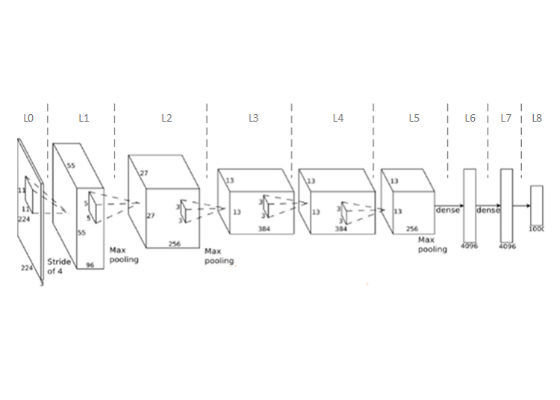Figure 5. AlexNet topology (credit: MIT*).

Table 1 provides additional details of the AlexNet architecture:

Layer
Type
Description
L0
Input image
Size: 227 x 227 x 3 (shown in diagram as 227 x 227 x 3)
L1
Convolution
Size: 55* x 55 x 96
96 filters, size 11 × 11
Stride 4
Padding 0
*Size = (N - F)/S + 1 = (227 - 11)/4 + 1 = 55
-
Max-pooling
Size: 27* x 27 x 96
96 filters, size 3 × 3
Stride 2
*Size = (N - F)/S + 1 = (55 – 3)/2 + 1 = 27
L2
Convolution
Size: 27 x 27 x 256
256 filters, size 5 x 5
Stride 1
Padding 2

-
Max-pooling
Size: 13* x 13 x 256
256 filters, size 3 × 3
Stride 2
*Size = (N - F)/S + 1 = (27 - 3)/2 + 1 = 13
L3
Convolution
Size: 13 x 13 x 384
384 filters, size 3 × 3
Stride 1
Padding 1

L4
Convolution
Size: 13 x 13 x 384
384 filters, size 3 × 3
Stride 1
Padding 1

L5
Convolution
Size: 13 x 13 x 256
256 filters, size 3 × 3
Stride 1
Padding 1

-
Max-pooling
Size: 6* x 6 x 256
256 filters, size 3 × 3
Stride 2
*Size = (N - F)/S + 1 = (13 - 3)/2 + 1 = 6
L6
Fully Connected
4096 neurons
L7
Fully Connected
4096 neurons
L8
Fully Connected
1000 neurons

Table 1. AlexNet layer descriptions.

A detailed description of convolutional neural networks and the AlexNet topology is beyond the scope of this tutorial, but the reader may find the following links useful if more information is required.

• Wikipedia - Convolutional Neural Networks
• Introduction to Convolutional Neural Nets
• Convolutional Neural Networks for Visual Recognition
• An Intuitive Explanation of Convolutional Neural Networks

Simple_Net Code Walkthrough

The source code presented below is essentially the same as the Simple_Net example contained in the repository, except it has been refactored to use the fully qualified Intel MKL-DNN types to enhance readability. This code implements the first layer (L1) of the topology.

1. Add include directive for the library header file:

#include "mkldnn.hpp"

2. Initialize the CPU engine as index 0:

auto cpu_engine = mkldnn::engine(mkldnn::engine::cpu, 0);

3. Allocate data and create tensor structures:

const uint32_t batch = 256;
std::vector<float> net_src(batch * 3 * 227 * 227);
std::vector<float> net_dst(batch * 96 * 27 * 27);
/* AlexNet: conv
* {batch, 3, 227, 227} (x) {96, 3, 11, 11} -> {batch, 96, 55, 55}
* strides: {4, 4}
*/
mkldnn::memory::dims conv_src_tz = {batch, 3, 227, 227};
mkldnn::memory::dims conv_weights_tz = {96, 3, 11, 11};
mkldnn::memory::dims conv_bias_tz = {96};
mkldnn::memory::dims conv_dst_tz = {batch, 96, 55, 55};
mkldnn::memory::dims conv_strides = {4, 4};
auto conv_padding = {0, 0};
std::vector<float> conv_weights(std::accumulate(conv_weights_tz.begin(),
conv_weights_tz.end(), 1, std::multiplies<uint32_t>()));
std::vector<float> conv_bias(std::accumulate(conv_bias_tz.begin(),
conv_bias_tz.end(), 1, std::multiplies<uint32_t>()));

4. Create memory for user data:

auto conv_user_src_memory = mkldnn::memory({{{conv_src_tz},
mkldnn::memory::data_type::f32,
mkldnn::memory::format::nchw}, cpu_engine}, net_src.data());
auto conv_user_weights_memory = mkldnn::memory({{{conv_weights_tz},
mkldnn::memory::data_type::f32, mkldnn::memory::format::oihw},
cpu_engine}, conv_weights.data());
auto conv_user_bias_memory = mkldnn::memory({{{conv_bias_tz},
mkldnn::memory::data_type::f32, mkldnn::memory::format::x}, cpu_engine},
conv_bias.data());

5. Create memory descriptors for convolution data using the wildcard any for the convolution data format (this enables the convolution primitive to choose the data format that is most suitable for its input parameters—kernel sizes, strides, padding, and so on):

auto conv_src_md = mkldnn::memory::desc({conv_src_tz},
mkldnn::memory::data_type::f32,
mkldnn::memory::format::any);
auto conv_bias_md = mkldnn::memory::desc({conv_bias_tz},
mkldnn::memory::data_type::f32,
mkldnn::memory::format::any);
auto conv_weights_md = mkldnn::memory::desc({conv_weights_tz},
mkldnn::memory::data_type::f32, mkldnn::memory::format::any);
auto conv_dst_md = mkldnn::memory::desc({conv_dst_tz},
mkldnn::memory::data_type::f32,
mkldnn::memory::format::any);

6. Create a convolution descriptor by specifying the algorithm, propagation kind, shapes of input, weights, bias, output, and convolution strides, padding, and padding kind:

auto conv_desc = mkldnn::convolution_forward::desc(mkldnn::prop_kind::forward,
mkldnn::convolution_direct, conv_src_md, conv_weights_md, conv_bias_md,
conv_dst_md, conv_strides, conv_padding, conv_padding,
mkldnn::padding_kind::zero);

7. Create a descriptor of the convolution primitive. Once created, this descriptor has specific formats instead of any wildcard formats specified in the convolution descriptor:

auto conv_prim_desc =
mkldnn::convolution_forward::primitive_desc(conv_desc, cpu_engine);

8. Create a vector of primitives that represents the net:

std::vector<mkldnn::primitive> net;

9. Create reorders between user and data if it is needed and add it to net before convolution:

auto conv_src_memory = conv_user_src_memory;
if (mkldnn::memory::primitive_desc(conv_prim_desc.src_primitive_desc()) !=
conv_user_src_memory.get_primitive_desc()) {
conv_src_memory = mkldnn::memory(conv_prim_desc.src_primitive_desc());
net.push_back(mkldnn::reorder(conv_user_src_memory, conv_src_memory));
}
auto conv_weights_memory = conv_user_weights_memory;
if (mkldnn::memory::primitive_desc(conv_prim_desc.weights_primitive_desc()) !=
conv_user_weights_memory.get_primitive_desc()) {
conv_weights_memory =
mkldnn::memory(conv_prim_desc.weights_primitive_desc());
net.push_back(mkldnn::reorder(conv_user_weights_memory,
conv_weights_memory));
}
auto conv_dst_memory = mkldnn::memory(conv_prim_desc.dst_primitive_desc());

10. Create convolution primitive and add it to net:

net.push_back(mkldnn::convolution_forward(conv_prim_desc, conv_src_memory,    conv_weights_memory, conv_user_bias_memory, conv_dst_memory));

11. Create a ReLU primitive and add it to net:

/* AlexNet: relu
* {batch, 96, 55, 55} -> {batch, 96, 55, 55}
*/
const double negative_slope = 1.0;
auto relu_dst_memory = mkldnn::memory(conv_prim_desc.dst_primitive_desc());
auto relu_desc = mkldnn::relu_forward::desc(mkldnn::prop_kind::forward,
conv_prim_desc.dst_primitive_desc().desc(), negative_slope);
auto relu_prim_desc = mkldnn::relu_forward::primitive_desc(relu_desc, cpu_engine);
net.push_back(mkldnn::relu_forward(relu_prim_desc, conv_dst_memory,
relu_dst_memory));

12. Create an AlexNet LRN primitive:

/* AlexNet: lrn
* {batch, 96, 55, 55} -> {batch, 96, 55, 55}
* local size: 5
* alpha: 0.0001
* beta: 0.75
*/
const uint32_t local_size = 5;
const double alpha = 0.0001;
const double beta = 0.75;
auto lrn_dst_memory = mkldnn::memory(relu_dst_memory.get_primitive_desc());
/* create lrn scratch memory from lrn src */
auto lrn_scratch_memory = mkldnn::memory(lrn_dst_memory.get_primitive_desc());
/* create lrn primitive and add it to net */
auto lrn_desc = mkldnn::lrn_forward::desc(mkldnn::prop_kind::forward,
mkldnn::lrn_across_channels,
conv_prim_desc.dst_primitive_desc().desc(), local_size,
alpha, beta);
auto lrn_prim_desc = mkldnn::lrn_forward::primitive_desc(lrn_desc, cpu_engine);
net.push_back(mkldnn::lrn_forward(lrn_prim_desc, relu_dst_memory,
lrn_scratch_memory, lrn_dst_memory));

13. Create an AlexNet pooling primitive:

/* AlexNet: pool
* {batch, 96, 55, 55} -> {batch, 96, 27, 27}
* kernel: {3, 3}
* strides: {2, 2}
*/
mkldnn::memory::dims pool_dst_tz = {batch, 96, 27, 27};
mkldnn::memory::dims pool_kernel = {3, 3};
mkldnn::memory::dims pool_strides = {2, 2};
auto pool_padding = {0, 0};
auto pool_user_dst_memory = mkldnn::memory({{{pool_dst_tz},
mkldnn::memory::data_type::f32,
mkldnn::memory::format::nchw}, cpu_engine}, net_dst.data());
auto pool_dst_md = mkldnn::memory::desc({pool_dst_tz},
mkldnn::memory::data_type::f32,
mkldnn::memory::format::any);
auto pool_desc = mkldnn::pooling_forward::desc(mkldnn::prop_kind::forward,
mkldnn::pooling_max, lrn_dst_memory.get_primitive_desc().desc(), pool_dst_md, pool_strides, pool_kernel, pool_padding, pool_padding,mkldnn::padding_kind::zero);
auto pool_pd = mkldnn::pooling_forward::primitive_desc(pool_desc, cpu_engine);
auto pool_dst_memory = pool_user_dst_memory;
if (mkldnn::memory::primitive_desc(pool_pd.dst_primitive_desc()) !=
pool_user_dst_memory.get_primitive_desc()) {
pool_dst_memory = mkldnn::memory(pool_pd.dst_primitive_desc());
}

14. Create pooling indices memory from pooling dst:

auto pool_indices_memory =
mkldnn::memory(pool_dst_memory.get_primitive_desc());

15. Create pooling primitive and add it to net:

net.push_back(mkldnn::pooling_forward(pool_pd, lrn_dst_memory,
pool_indices_memory, pool_dst_memory));

16. Create reorder between internal and user data if it is needed and add it to net after pooling:

if (pool_dst_memory != pool_user_dst_memory) {
net.push_back(mkldnn::reorder(pool_dst_memory, pool_user_dst_memory));
}

17. Create a stream, submit all the primitives, and wait for completion:

mkldnn::stream(mkldnn::stream::kind::eager).submit(net).wait();

18. The code described above is contained in the simple_net() function, which is called in main with exception handling:

int main(int argc, char **argv) {
try {
simple_net();
}
catch(mkldnn::error& e) {
std::cerr << "status: " << e.status << std::endl;
std::cerr << "message: " << e.message << std::endl;
}
return 0;
}

Conclusion

Part 1 of this tutorial series identified several resources for learning about the technical preview of Intel MKL-DNN. Detailed instructions on how to install and build the library components were also provided. In this paper (Part 2 of the tutorial series), information on how to configure the Eclipse integrated development environment to build the C++ code sample was provided, along with a code walkthrough based on the AlexNet deep learning topology. Stay tuned as Intel MKL-DNN approaches production release.

For more such intel IoT resources and tools from Intel, please visit the Intel® Developer Zone

Source:https://software.intel.com/en-us/articles/intel-mkl-dnn-part-2-sample-code-build-and-walkthrough

Promotion
advertisements
ASK DIGIT

#### Recent Questions

ASCII code?
Vivek Bhatt
Sept 3, 2014
Responses 2
vishal Pallerla
Sept 3, 2014
Hina
Sept 7, 2014
Comments
Be the first one to post the comment
Post a New Comment
You must be signed in to post a comment
advertisements